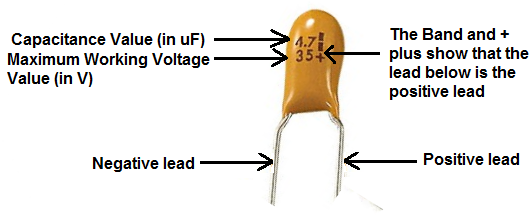﻿ How to Read a Tanatalum Capacitor ﻿# How to Read a Tantalum CapacitorIn this article, we go over how to read and decipher the values on tantalum capacitors so that we can understand and decode their values.

Tantalum capacitors usually come with 2 numbers on them along with a long band a positive plus sign ("+"). These are all there to help us discover what value of capacitance the capacitor is, its maximum working voltage, and which lead is positive.

Below is a diagram of a tantalum capacitor with the meanings of its components labeled:The topmost value to the left is the value of the capacitance of the capacitor in unit microfarads (uF). In the example above, this value is 4.7, so the capacitor has a capacitance of 4.7uF.

The value right below this is the maximum working voltage of the capacitor. The maximum working voltage is the maximum amount of voltage which can be dropped across the capacitor in a circuit. This value is in unit volts (V). In the capacitor example above, the capacitor has a MWV of 35V. This means that in a circuit, a designer should make sure that no more than 35V is dropped across the capacitor. If the capacitors receives more than 35V, then it can be either partially or totally destroyed and, thus, rendered useless in a circuit. The voltage applied across the cap should never exceed this maximum working voltage value.

To the right of the capacitor is a long band a positive plus sign ("+"). This long band and + sign denote that this side contains the positive lead. So the other side, then, is the negative lead. To find out more about deciphering the positive and negative leads of tantalum capacitors, see Tantalum Capacitor Polarity Markings.

And these are the key components to reading tantalum capacitor values.

Related Resources

What are Tantalum Capacitors?

Types of Capacitors